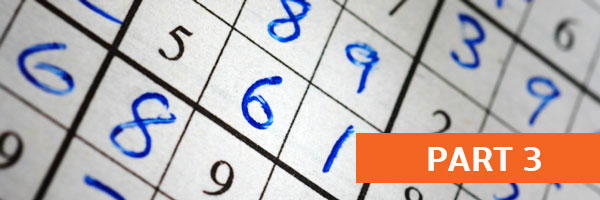# How to Solve Sudoku Puzzles – A Complete Walkthrough, Part 3As shown in the figure below, there is a column (outlined in green) that has only 3 spaces missing. We know from looking at the existing numbers (1, 3, 4, 5, 8, 9) that the missing numbers are 2, 6 and 7. If we look to the nearby squares, we can see a 2 in the middle left square (circled in red) and in the bottom row of the upper right square (circled in red).

This means that the top space of the green column must contain 2 (marked in blue).

Now that we’ve filled in the top empty space with number 2, we can look at the two remaining empty spaces in the green column. We know that they must contain a 6 and a 7. In the upper right square, there is a 7 (circled in red) in the bottom row, so that means the empty space in the lower left corner of the top center square must contain a 6 (marked in blue). This leaves us with a 7 as the last remaining number to be placed in the green column.

Now that we’ve completed the green column, we can try to keep the momentum going in the top center square. There are only three empty spaces remaining (now that we’ve placed the 2 and 6, in blue), and we know that the numbers must be 3, 4 and 5. To the left and the right, we can see two number 5s (circled in red) which means that the number 5 must be in the upper left space of the top center square (marked in blue).

Now there are only two spaces left, for number 3 and 4. We can see a 4 in the upper left square (circled in red) so that means the blank space in the center of the top square must be number 3, and the lower right space must be number 4 (both marked in blue). Suddenly, we’ve solved a whole column and another whole square at the top center of the grid, as shown below. Now let’s look at the row and column outlined in green that only have two empty spaces remaining, and let’s see what other empty spaces we can fill.

The central column (in green) has only two spaces left, and they must contain a 2 and a 6.

In the center-right square, there is a 6 (circled in red) which shows us that we can eliminate 6 as being in the top empty space of the column – so that space must contain 2 (marked in blue). Also, this means that the only remaining space in the green column must contain 6 (marked in blue).

Now, looking to the green row, that same number 6 (circled in red) also shows us that we can eliminate the 6 from being in the far-right empty space of the green row – so that means a 1 must go there. (There is also a 1 circled in red in the lower right square, which eliminates that left center space in the top right square from containing 1 – so there are plenty of clues here.)

Now we have filled almost 2/3 of the Sudoku grid! Let’s see what we can solve in our next article in the series.

Please read part 4 of this series on how to solve Sudoku puzzles, using this actual game as an example. (Coming soon!)